# Mysql到底是不是一颗数据库？热

gen 发布于 2011/04/27 10:50

/*[9:51:43][11232 ms]*/ SELECT * FROM userinfo WHERE id=99999  LIMIT 0, 1000;

/*[9:54:29][ 109 ms]*/ SELECT * FROM userinfo WHERE id>99999 AND age!=280 AND NAME='testuser' LIMIT 1 ;

/*[9:55:31][11560 ms]*/ SELECT * FROM userinfo WHERE id=9999999 AND age!=280 AND NAME='testuser' LIMIT 1 ;

/*[10:01:07][170337 ms]*/ ALTER TABLE `userinfo` ADD PRIMARY KEY ( `id` )  ;

/*[10:10:28][283063 ms]*/ ALTER TABLE `userinfo` ADD INDEX age ( `age` )  ;

/*[10:19:13][355651 ms]*/ ALTER TABLE `userinfo` ADD INDEX usearch ( `id`,`age` )  ;

/*[10:21:12][  16 ms]*/ SELECT * FROM userinfo WHERE id=99999  LIMIT 0, 1000;

/*[10:21:40][  47 ms]*/ SELECT * FROM userinfo WHERE id>99999 AND age!=280 AND NAME='testuser' LIMIT 1 ;

/*[10:22:30][   0 ms]*/ SELECT * FROM userinfo WHERE id=9999999 AND age!=280 AND NAME='testuser' LIMIT 1 ;

/*[10:36:40][  31 ms]*/ SELECT * FROM userinfo WHERE id=99999  LIMIT 0, 1000;

/*[10:37:41][  47 ms]*/ SELECT * FROM userinfo WHERE id>99999 AND age!=280 AND NAME='testuser' LIMIT 1 ;

/*[10:38:11][  31 ms]*/ SELECT * FROM userinfo WHERE id=9999999 AND age!=280 AND NAME='testuser' LIMIT 1 ;

```/*
本程序用来测试数据库的压力
*/

set_time_limit(0);

/* 数据库链接 */
\$db = mysql_connect('localhost', 'root', 'aaaaaa');
mysql_select_db('dbtest');

\$start_time = time();

/* 插入数据 */
/*
\$x = 0;
while(\$x < 10000000)
{
\$sql = "insert into userinfo values";
\$max = \$x + 3000;
\$age = rand(1, 100);
for(\$x; \$x < \$max; \$x++)
{
\$sql .= "('{\$x}', 'testuser', '{\$age}'),";
}

\$sql = substr(\$sql, 0, -1);
\$res = mysql_query(\$sql);
if (\$res == true)
{
echo "{\$x}-ok@<br />";
}

\$x = \$max;
}
*/
\$x = 10000000;
while(\$x < 20000000)
{
\$sql = "insert into userinfo values";
\$max = \$x + 3000;
\$age = rand(1, 100);
for(\$x; \$x < \$max; \$x++)
{
\$sql .= "('{\$x}', 'testuser', '{\$age}'),";
}

\$sql = substr(\$sql, 0, -1);
\$res = mysql_query(\$sql);
if (\$res == true)
{
echo "{\$x}-ok@<br />";
}

\$x = \$max;
}

\$use_time = time() - \$start_time;
echo "插入1000万条数据共用时：".\$use_time."秒";```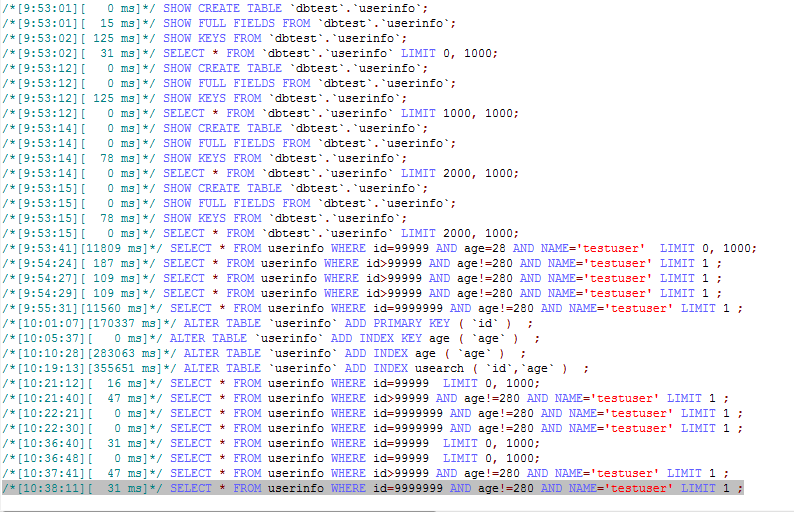0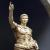0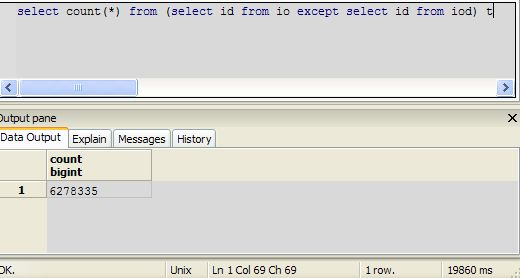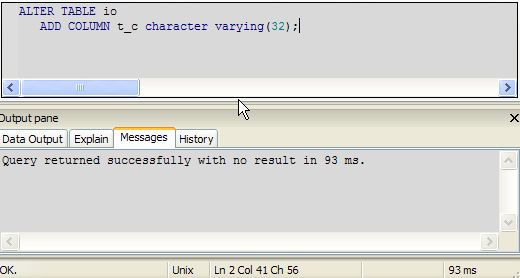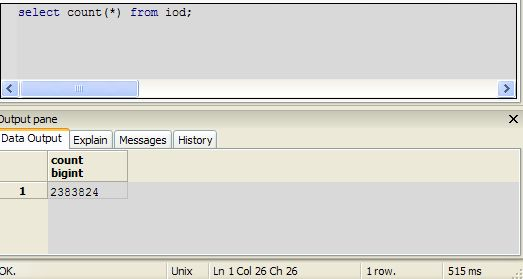0alter index usearch.

0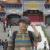MySQL性能上比不过Oracle的是必然的，不然Oracle就没人买了，但是对于中小项目来说MySQL的简单轻量及免费的特点还是比较适合的。

0#### 引用来自#8楼“戏水”的帖子

MySQL性能上比不过Oracle的是必然的，不然Oracle就没人买了，但是对于中小项目来说MySQL的简单轻量及免费的特点还是比较适合的。

0Pgsql没用过，不过我相信应该不错，但网上的使用经验和资料相对MySQL来说太少了，大部分的人都有求安逸的思想，如果没有明显的压倒性优势也就懒得换了

0#### 引用来自#10楼“戏水”的帖子

Pgsql没用过，不过我相信应该不错，但网上的使用经验和资料相对MySQL来说太少了，大部分的人都有求安逸的思想，如果没有明显的压倒性优势也就懒得换了